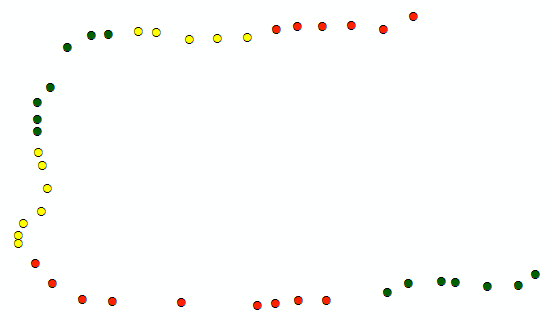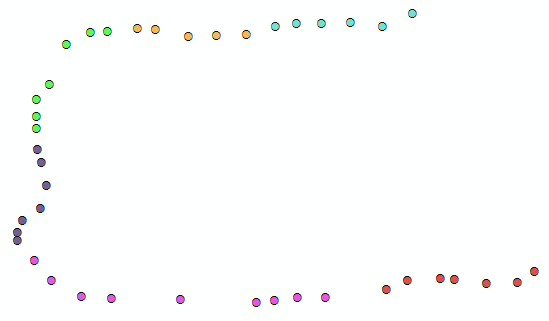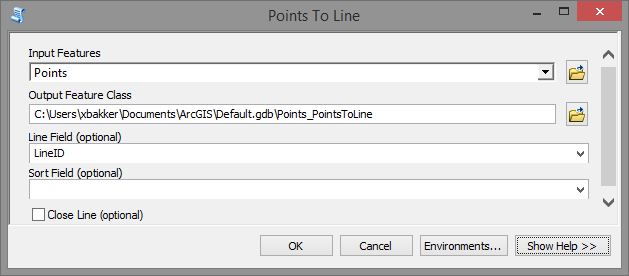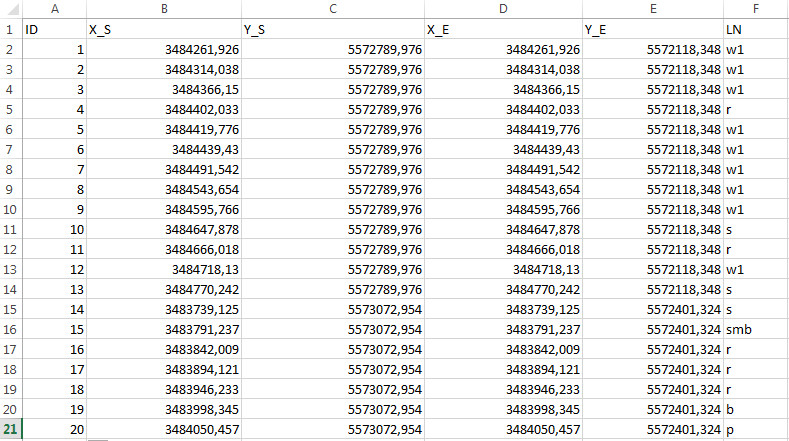# Create a line from points using attributes

10918
11
05-04-2016 06:25 AMNew Contributor II

Hello all,

I have a field in my point layer that contains values, for example, go something like the following: 1,1,1,1,1,1,3,3,3,3,2,2,2,2,3,3,3,3,3,1,1,1,1

I am looking to create separate line features for each set of unique values.  Using the example above, one line feature would be connecting the first 6 points that contain 1, the next line feature would contain the 4 points that contain 3, etc.  I initially tried using the Points to Line geoprocessing tool and populate this field in my point layer in the Line Field.  However my results looked like example 1 (See attached LineExample1.PNG).  I am looking for whenever there is a different value to a point, the lines do not join (see attached LineExample2.PNG).

So in short, I am looking to do LineExample2 and not LineExample1.  Any help would be greatly appreciated!

Tags (2)
1 Solution

Accepted SolutionsbyEsri Esteemed Contributor

From what I'm seeing, probably the easiest method would be to create a new field with the LineID and fill it like this:Pre-Logic  Script Code:

```line_id = 1
prev_class = 1
def getLineID(val):
global prev_class
global line_id
if val == prev_class:
return line_id
else:
line_id += 1
prev_class = val
return line_id```

So instead of using the CLASS field to generate the lines:You will have the LineID field with a unique value for each group of sequential CLASS values:Use the LineID field when executing the Point to Line tool in the Line Field option:Which will generate the lines correctly:11 RepliesbyMVP Frequent Contributor

It sounds like the lines to be generated fall into a few categories, but there are many more lines than categories (i.e. multiple lines desired for each category), so just creating the lines by the category is tripping things up.

As a workaround, is there any way to identify before running Points to Line which points are grouped together?  If there is, one could then add a new field to your data, then calculate a new unique ID for each point in an identified "line group", then run Points to Line using the new field for the Line Field parameter so as to get the lines as you want.  You would still have the original values in your input points to Spatial Join to the lines if need be so one could get the original categories assigned.

Chris Donohue, GISPRegular Contributor III

Not sure if this will work for you Aggregate Points—Help | ArcGIS for DesktopbyMVP Legendary Contributor

From figure 2, you have a multipart shape which, in your case, consists of two spatially separated lines sharing one record in the table.  How was this generated?  I have seen that when converting shapes to numpy arrays with the explode option.  In such cases it is possible to reassemble the shapes appropriately.  If you have no clue what I am talking about ... nevermind... an explanation probably wouldn't helpOccasional Contributor III

When you use Point To line tool in (Data Management tool - Features), you can create continues line through same value in specific field, using "Line Field" option in that tool.

BUT

if you make that, your line will be through long distance and you will have  (LineExample1) as your picture.

So

the Solution for this problem that:

you should create other field for classify each group, to use that field in (Line Field ) option in Point To Line Tool.

But

How Can I create new class for each group .

You can Make that using Buffer tool.

Buffer Tool????

yes Buffer tool for your point feature class and make the buffer distance for ( maximum distance between closest point for each group not each class)

that will make a polygon contains a group of points for one Line .

You can do that if you make dissolve Buffer. using the field that has original classes for each group, and choose that field in Dissolve Field option in Buffer tool.

then you should explode feature using Multipart To Singlepart Tool.

Now

You have to select each polygon and select points within that polygon and that points must have same class of polygon, because the polygon may be large to contain other class of points. After selection you should make Calculate Field with unique value .

You Can make that with Model builder and use iteration and make the unique value calculation in the number of iteration ( %n%).

After model finish, You can use Point To line tool, and  choose the new classes field that you calculated in model builder in  "Line Field" option .

and you will have the lines as (LineExample2) picture.

thanksbyEsri Esteemed Contributor

From what I'm seeing, probably the easiest method would be to create a new field with the LineID and fill it like this:Pre-Logic  Script Code:

```line_id = 1
prev_class = 1
def getLineID(val):
global prev_class
global line_id
if val == prev_class:
return line_id
else:
line_id += 1
prev_class = val
return line_id```

So instead of using the CLASS field to generate the lines:You will have the LineID field with a unique value for each group of sequential CLASS values:Use the LineID field when executing the Point to Line tool in the Line Field option:Which will generate the lines correctly:New Contributor II

Thanks all for your suggestions.  They were all extremely useful.  I ended up using the latest post and then joined the output using the LineID's in both the point and line layers to get the CLASS field into the outputted line layer.New Contributor

Hello at all,

I have a script using start and  (S_X, S_Y) end points (E_X, E_Y) as coordinates from an excel table in order to create lines, it looks like the following:

``import arcpy  in_rows = arcpy.SearchCursor(r"D:\Temp\Lines.xls\Sheet1\$") point = arcpy.Point()array = arcpy.Array() featureList = []cursor = arcpy.InsertCursor(r"D:\Temp\Lines.shp")feat = cursor.newRow()for in_row in in_rows:    # Set X and Y for start and end points    point.X = in_row.S_X     point.Y = in_row.S_Y     array.add(point)    point.X = in_row.E_X     point.Y = in_row.E_Y     array.add(point)        # Create a Polyline object based on the array of points    polyline = arcpy.Polyline(array)    # Clear the array for future use    array.removeAll()    # Append to the list of Polyline objects    featureList.append(polyline)    # Insert the feature    feat.shape = polyline     cursor.insertRow(feat)del featdel cursor``It works well for my purpose, but I'd need to carry over information of another coloumn ``

`(see excel table jpg, the LN coloumn), so that the`

`resulting lines have this information as a coloumn of the attribute table.`
`Any ideas?`

````byEsri Esteemed Contributor

Hi 1tiga11 ,

You will need to create the output field first and write the result to the output feature.

I notice that you are using the arcpy.InsertCursor. If you have 10.1 or higher it is better to use the arcpy.da.InsertCursor instead.

Kind regards, XanderNew Contributor

Dear Xander,

could you give me an example how this should be done?